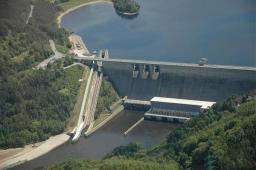# Simultaneously 4479

A worker would fill the tank with one inlet in 36 minutes, the other in 45 minutes. How long will it take to fill the tank if the water flows through the first inlet for 9 minutes and then both simultaneously?

t =  24 min

### Step-by-step explanation:

t/36+(t-9)/45=1

9t = 216

t = 216/9 = 24

t = 24

Our simple equation calculator calculates it.Did you find an error or inaccuracy? Feel free to write us. Thank you!

Tips for related online calculators
Do you have a linear equation or system of equations and looking for its solution? Or do you have a quadratic equation?